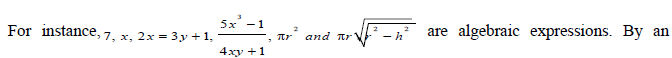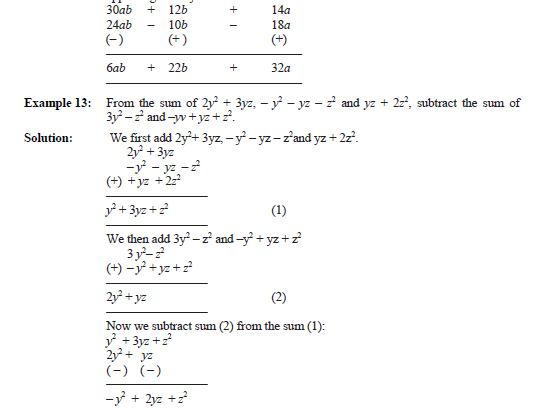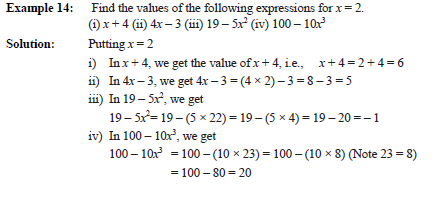# CBSE Class 6 Mathematics Algebra Chapter Notes

Download CBSE Class 6 Mathematics Algebra Chapter Notes in PDF format. All Revision notes for Class 6 Algebra have been designed as per the latest syllabus and updated chapters given in your textbook for Algebra in Standard 6. Our teachers have designed these concept notes for the benefit of Grade 6 students. You should use these chapter wise notes for revision on daily basis. These study notes can also be used for learning each chapter and its important and difficult topics or revision just before your exams to help you get better scores in upcoming examinations, You can also use Printable notes for Class 6 Algebra for faster revision of difficult topics and get higher rank. After reading these notes also refer to MCQ questions for Class 6 Algebra given our website

## Class 6 Algebra Revision Notes

Class 6 Algebra students should refer to the following concepts and notes for Algebra in standard 6. These exam notes for Grade 6 Algebra will be very useful for upcoming class tests and examinations and help you to score good marks

### Notes Class 6 Algebra

CBSE Class 6 Algebra Chapter Concepts. Learning the important concepts is very important for every student to get better marks in examinations. The concepts should be clear which will help in faster learning. The attached concepts made as per NCERT and CBSE pattern will help the student to understand the chapter and score better marks in the examinations.

.11.1 Beginning of Algebra

It is said that algebra as a branch of Mathematics began about 1550 BC, i.e. more than 3500 years ago, when people in Egypt started using symbols to denote unknown numbers. Around 300 BC, use of letters to denote unknowns and forming expressions from them was quite common in India. Many great Indian mathematicians, Aryabhatt (born 476AD), Brahmagupta (born 598AD), Mahavira (who lived around 850AD) and Bhaskara II (born 1114AD) and others, contributed a lot to the study of algebra. They gave names such as Beeja, Varna etc. to unknowns and used first letters of colour names [e.g., ka from kala (black), nee from neela (blue)] to denote them. The Indian name for algebra, Beejaganit, dates back to these ancient Indian mathematicians. The word ‘algebra’ is derived from the title of the book, ‘Aljebar w’al almugabalah’, written about 825AD by an Arab mathematician, Mohammed Ibn Al Khowarizmi of Baghdad.

11.2 Algebra

Algebra is about finding the unknown or it is about putting real life problems into equations and then solving them. Unfortunately many textbooks go straight to the rules, procedures and formulas, forgetting that these are real life problems being solved. A branch of mathematics that substitutes letters for numbers. An algebraic equation represents a scale, what is done on one side of the scale with a number is also done to the other side of the scale. The numbers are the constants. Algebra can include real numbers, complex numbers,
matrices, vectors etc.

11.3 Why Do I Need Algebra?

Only you can answer this question. I've always said math is an opportunity gateway and you can't get to higher Maths without taking algebra. Algebra develops your thinking, specifically logic, patterns, problem solving, deductive and inductive reasoning. The more math you have, the greater the opportunity for jobs in engineering, actuary, Physics, programming etc. Higher math is often an important requirement for entrance to college or universities. Ultimately, you need to do your own homework to determine if your goals mean sticking it out in math, however, you can never go wrong if you do

11.4 Algebraic Expression
An algebraic Expressions is an expression formed from any combination of numbers and variables by using the operations of addition, subtraction, multiplication, division, exponentiation (raising to powers), or extraction of roots.algebraic expression in certain variables, we mean an expression that contains only those variables, and by a constant, we mean an algebraic expression that contains no variables at all. If numbers are substituted for the variables in an algebraic expression, the resulting number is called the value of the expression for these values of the variables.

11.5 Algebraic Terms

The basic unit of an algebraic expression is a term. In general, a term is either a number or a product of a number and one or more variables. Below is the term –3ax.11.6 How to Form Algebraic Expressions?
Example 1: Sarita has some marbles. Ameena has 10 more. Appu says that he has 3 more marbles than the number of marbles Sarita and Ameena together have. How do you get the number of marbles that Appu has?

Solution: Since it is not given how many marbles Sarita has, we shall take it to be x. Ameena then has 10 more, i.e., x + 10. Appu says that he has 3 more marbles than what Sarita and Ameena have together. So we take the sum of the numbers of Sarita’s marbles and Ameena’s marbles, and to this sum add 3, that is, we take the sum of x, x + 10 and 3.

Example 2: Ramu’s father’s present age is 3 times Ramu’s age. Ramu’s grandfather’s age is 13 years more than the sum of Ramu’s age and Ramu’s father’s age. How do you find Ramu’s grandfather’s age?

Solution: Since Ramu’s age is not given, let us take it to be y years. Then his father’s age is 3y years. To find Ramu’s grandfather’s age we have to take the sum of Ramu’s age (y) and his father’s age (3y) and to the sum add 13, that is, we have to take the sum of y, 3y and 13.

Example 3: In a garden, roses and marigolds are planted in square plots. The length of the square plot in which marigolds are planted is 3 meters greater than the length of the square plot in which roses are planted. How much bigger in area is the marigold plot than the rose plot?

Solution: Let us take l meters to be length of the side of the rose plot. The length of the side of the marigold plot will be (l + 3) meters. Their respective areas will be l2 and (l+ 3) 2. The difference between (l+ 3) 2 and l2 will decide how much bigger in area the marigold plot is. In all the three situations, we had to carry out addition or subtraction of algebraic expressions. There are a number of real life problems in which we need to use expressions and do arithmetic operations on them. In this section, we shall see how algebraic expressions are added and subtracted.

11.7 Addition and Subtraction of Like Terms
The simplest expressions are monomials. They consist of only one term. To begin with we shall learn how to add or subtract like terms.

Example 4: Add 3x and 4x.

Solution: To add 3x and 4x, keep the variable part as it is. Just add the co-efficients of each like term. i.e., 3x + 4x = (3 × x) + (4 × x)
= (3 + 4) × x (using distributive law) = 7 × x = 7x or 3x + 4x = 7x

Example 5: Add 8xy, 4xy and 2xy

Solution: 8xy + 4xy + 2xy = (8 + 4 + 2) × xy = 14 × xy = 14xy or 8xy + 4xy + 2xy = 14xy

Example 6 : Subtract 4n from 7n.

Solution: 7n – 4n = (7 × n) – (4 × n) = (7 – 4) × n = 3 × n = 3n or 7n – 4n = 3n

Example 7: Subtract 5ab from 11ab.

Solution: 11ab – 5ab = (11 – 5) ab = 6ab

Thus, the sum of two or more like terms is a like term with a numerical coefficient equal to the sum of the numerical coefficients of all the like terms. Similarly, the difference between two like terms is a like term with a numerical coefficient equal to the difference between the numerical coefficients of the two like terms.

Note: Unlike terms cannot be added or subtracted the way like terms are added or subtracted. We have already seen examples of this, when 5 is added to x, we write the result as (x + 5). Observe that in (x + 5) both the terms 5 and x are retained. Similarly, if we add the unlike terms 3xy and 7, the sum is 3xy + 7. If we subtract 7 from 3xy, the result is 3xy – 7.

11.8 Adding and Subtracting General Algebraic Expressions

Let us take some examples:

Example 8: Add 3x + 11 and 7x – 5

Solution:

(3x + 11) + (7x – 5) = 3x + 11 + 7x – 5

= 3x + 7x + 11 – 5 (rearranging terms)

= (3x + 7x) + (11 – 5) (grouping like terms) = 10x + 6

Hence, 3x + 11 + 7x – 5 = 10x + 6

Example 9: Add 3x + 11 + 8z and 7x – 5.

Solution:

(3x + 11 + 8z) + (7x – 5) = 3x + 11 + 8z + 7x – 5\

= (3x + 7x) + (11 – 5) + 8z (grouping like terms) = 10 x + 6 + 8z

Therefore, the sum = 10x + 6 + 8z

Example 10: Subtract a – b from 3a – b + 4

Solution: (3a – b + 4) – (a – b) = 3a – b + 4 – a + b

= (3a – a) + (b – b) + 4 (grouping like terms )

= (3 – 1) a + (1 – 1) b + 4 (using Distributive law ) = 2a + (0) b + 4 = 2a + 4

(or) 3a – b + 4 – (a – b) = 2a + 4

Example 11: Collect like terms and simplify the expression:

12m2 – 9m + 5m – 4m2– 7m + 10

Solution: Rearranging terms, we have

12m2 – 4m2+ 5m – 9m – 7m + 10 = (12 – 4) m2 + (5 – 9 – 7) m + 10

12m 2 – 4m 2+ 5m – 9m – 7m + 10 = 8m2 + (– 4 – 7) m + 10

= 8m2 + (–11) m + 10 = 8m2 – 11m + 10

Example 12: Subtract 24ab – 10b – 18a from 30ab + 12b + 14a.

Solution:

30ab + 12b + 14a – (24ab – 10b – 18a)

= 30ab + 12b + 14a – 24ab + 10b + 18a

= 30ab – 24ab + 12b + 10b + 14a + 18a

= 6ab + 22b + 32a

Alternatively, we write the expressions one below the other with the like terms appearing exactly below like terms as:9. Finding the Value of an Expression

We know that the value of an algebraic expression depends on the values of the variables forming the expression. There are a number of situations in which we need to find the value of an expression, such as when we wish to check whether a particular value of a variable satisfies a given equation or not. We find values of expressions, also, when we use formulas from geometry and from everyday mathematics. For example, the area of a square is l2, where l is the length of a side of the square. If l = 5 cm., the area is 52 cm2 or 25 cm2; if the side is 10 cm, the area is 102cm2or 100 cm2 and so on. We shall see more such examples in the next section.11.10 Simple Equation

Equation: Any equation is a condition on a variable. It is satisfied only for a definite value of the variable

Note: An equation has an equal sign (=) between its two sides. The equation says that the value of the left hand side (LHS) is equal to the value of the right hand side (RHS). If the LHS is not equal to the RHS, we do not get an equation. For example , The statement 2n is greater than 10, i.e. 2n > 10 is not an equation. Similarly, the statement 2n is smaller than 10 i.e. 2n < 10 is not an equation.

Solution of an Equation: The value of the variable in an equation which satisfies the equation is called a solution to the equation. Thus, n = 5 is a solution to the equation 2 n = 10.

11.11 Introduction

In this section we shall look at some simple equations and the methods used to find their solution. There are four basic rules:

Rule 1: An equal quantity may be added to both sides of an equation.

Rule 2: An equal quantity may be subtracted form both sides of an equation.

Rule 3: An equal quantity may multiply both sides of an equation.

Rule 4: An equal non-zero quantity may divide both sides of an equation.

## Tags:

Click for more Algebra Study Material
 CBSE Class 6 Mathematics Algebra Chapter Notes CBSE Class 6 Mathematics Algebra Notes Set A CBSE Class 6 Mathematics Algebra Notes Set B

## Latest NCERT & CBSE News

Read the latest news and announcements from NCERT and CBSE below. Important updates relating to your studies which will help you to keep yourself updated with latest happenings in school level education. Keep yourself updated with all latest news and also read articles from teachers which will help you to improve your studies, increase motivation level and promote faster learning

### Online courses for classes XI and XII offered by NCERT

Ministry of Education (MoE), Government of India has launched a platform for offering Massive Open Online Courses (MOOCs) that is popularly known as SWAYAM (Study Webs of Active learning for Young Aspiring Minds) on 9 th July, 2017. NCERT now offers online courses for...

### Pariksha Pe Charcha 2022

The 5th edition of Pariskhas Pe Charcha the unique interactive program of Hon’ble Prime Minister with students teaches and parents will be held through virtual mode in February, 2022. In order to select participants who will be featured in Pariksha Pe Charcha programme...

### National Youth Day and Birth Anniversary of Swami Vivekananda

Ministry of Education, Govt. of India vide D.O No. 12-4/2021-IS.4 dated 04.01.2022 intimated that 12 January 2022 will be celebrated as “National Youth Day” and “Birth Anniversary of Swami Vivekananda”.   All Schools affiliated to CBSE may celebrate 12 January 2022 as...

### Heritage India Quiz 2021 2022

CBSE Heritage India Quiz is conducted every year to raise the awareness about the preserving human heritage, diversity and vulnerability of the India's built monuments and heritage sites. It is an attempt of the Board to motivate the future generations of this country...

### Surya Namaskar Project on 75th Anniversary of Independence Day

Ministry of Education, Govt of India vide letter No. F.No. 12-5/2020-IS-4 dated 16.12.2021 has intimated that under the banner Azadi ka Amrit Mahotsav the National Yogasanasports Federation has decided to run a project of 750 million Surya Namaskar from 01 January 2022...